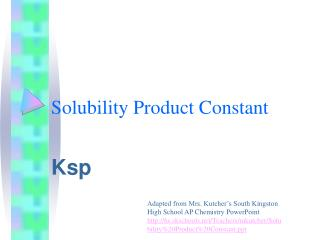Download PresentationSolubility Product Constant

# Solubility Product Constant

Download Presentation## Solubility Product Constant

- - - - - - - - - - - - - - - - - - - - - - - - - - - E N D - - - - - - - - - - - - - - - - - - - - - - - - - - -
##### Presentation Transcript

1. Solubility Product Constant Ksp Adapted from Mrs. Kutcher’s South Kingston High School AP Chemistry PowerPoint http://hs.skschools.net/Teachers/mkutcher/Solubility%20Product%20Constant.ppt

2. Ksp, the solubility-product constant. An equilibrium can exist between a partially soluble substance and its solution:

3. For example: BaSO4 (s)  Ba2+ (aq) + SO42- (aq) • When writing the equilibrium constant expression for the dissolution of BaSO4, we remember that the concentration of a solid is constant. The equilibrium expression is therefore: K = [Ba2+][SO42-] K = Ksp, the solubility-product constant. Ksp = [Ba2+][SO42-]

4. The Solubility Expression AaBb(s)  aAb+ (aq) + bBa- (aq) Ksp = [Ab+]a [Ba-]b Example: PbI2 (s)  Pb2+ + 2 I- Ksp = [Pb2+] [I-]2 • The greater the ksp the more soluble the solid is in H2O.

5. Solubility and KspThree important definitions: • solubility: quantity of a substance that dissolves to form a saturated solution • molar solubility: the number of moles of the solute that dissolves to form a liter of saturated solution • Ksp (solubility product): the equilibrium constant for the equilibrium between an ionic solid and its saturated solution

6. Calculating Molar Solubility Calculate the molar solubility of Ag2SO4 in one liter of water. Ksp =1.4 x 10-5 Ag2SO4 2Ag+ + SO42- Initial 0 0 Change +2x +x Equilb 2x x Ksp = [Ag+]2[SO42-] = (2x)2(x)= 1.4 x 10-5 X = 1.5 x 10-2 mol Ag2SO4 /L (molar solubility)

7. Will a Precipitation Occur? If 1.00 mg of Na2CrO4 is added to 225 ml of 0.00015 M AgNO3, will a precipitate form? Ag2CrO4 (s)  2Ag+ + CrO42- Determine the initial concentration of ions. Ag+ = 1.5 x 10-4 CrO42- = 1.00 x 10-3 g / MM = 6.17 x 10-6 mol CrO42-/.225 L = 2.74 x 10-5 M

8. Will a Precipitation Occur? • Compare the initial concentration to the solubility product constant Initial concentration of ions: (Ag+)2 (CrO42-) (1.5 x 10-4)2 (2.74 x 10-5 M)= 6.2 x 10-13 Ag2CrO4 Ksp = 1.1 x 10-12 • No precipitation will occur because the initial concentration is less than the Ksp.

9. Predicting if Precipitation Occurs Step 1: write the balanced equilibrium: Ag2CrO4(s)  2Ag+(aq) + CrO42–(aq) Step 2: Write the Ksp equation: Ksp = [Ag+]2[CrO42–] = 1.2 x 10–12 Step 3: Determine the initial concentration of ions [Ag+]2[CrO42–] = [4.8 x 10–5]2[3.4 x 10–4] = 7.8 x 10–13 ion product is less than Ksp, thus no precipitate will form (more could be dissolved)

10. Common ion Effect PbI2(s) Pb2+(aq) + 2I–(aq) Common ion: “The ion in a mixture of ionic substances that is common to the formulas of at least two.” Common ion effect: “The solubility of one salt is reduced by the presence of another having a common ion”

11. Common Ion Effect • Thus the solubility of the PbI2 is reduced by the presence of the NaI. Ksp of PbI2 = 7.9 x 10-9, so the molar solubility is 7.9 x 10-9 = (x)(2x)2 = 4x3 X = 1.3 x 10-3 Which is much greater than 7.9 x 10-7 when 0.10 M NaI is in solution.

12. Common Ion Effect • When two salt solutions that share a common ion are mixed the salt with the lower ksp will precipitate first. Example: AgCl ksp = [Ag+][Cl-] = 1.6 x10-10 [Ag+][Cl-] = 1.6 x 10-10 X2 = 1.6 x 10-10 X =[Ag+] = [Cl-] = 1.3 x 10-5 M

13. Common Ion Effect Add 0.10 M NaCl to a saturated AgCl solution. [Cl-] = 0.10 (common ion) [Ag+][Cl-] = 1.6 x 10-10 [Ag+][0.10 + x] = 1.6 x 10-10 (x is small) [Ag+]= 1.6 x 10-10/ 0.10 M [Ag+]= 1.6 x 10-9 • [Ag+] = 1.3 x 10-5 from the previous slide So, some AgCl will precipitate when the NaCl is added because the molar solubility of the solution is now less than the that of AgCl alone.

14. Will a Precipitation Occur? If 1.00 mg of Na2CrO4 is added to 225 ml of 0.00015 M AgNO3, will a precipitate form? Ag2CrO4 (s)  2Ag+ + CrO42- Determine the initial concentration of ions. Ag+ = 1.5 x 10-4 CrO42- = 1.00 x 10-3 g / MM = 6.17 x 10-6 mol CrO42-/.225 L = 2.74 x 10-5 M

15. Will a Precipitation Occur? • Compare the initial concentration to the solubility product constant Initial concentration of ions: (Ag+)2 (CrO42-) (1.5 x 10-4)2 (2.74 x 10-5 M)= 6.2 x 10-13 Ag2CrO4 Ksp = 1.1 x 10-12 • No precipitation will occur because the initial concentration is less than the Ksp.

16. Predicting if Precipitation Occurs Step 1: write the balanced equilibrium: Ag2CrO4(s)  2Ag+(aq) + CrO42–(aq) Step 2: Write the Ksp equation: Ksp = [Ag+]2[CrO42–] = 1.2 x 10–12 Step 3: Determine the initial concentration of ions [Ag+]2[CrO42–] = [4.8 x 10–5]2[3.4 x 10–4] = 7.8 x 10–13 ion product is less than Ksp, thus no precipitate will form (more could be dissolved)